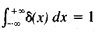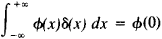# Delta Function

(redirected from Unit impulse function)

## delta function

[′del·tə ‚fəŋk·shən]
(mathematics)
A distribution δ such that is ƒ(x). Also known as Dirac delta function; Dirac distribution; unit impulse.

## Delta Function

(also δ function, Dirac delta function, or δ(x)), a symbol used in mathematical physics in solving problems in which there are point magnitudes (for example, point load and point charge). The delta function can be defined as the density of the distribution of masses, for which a unit mass is concentrated at the point x = 0, while the mass at all other points is equal to zero. Therefore, it is assumed that δ(x) = 0, when x ≠ 0 and δ(0) = ∞, while(“infinite splash” of a “unit intensity”). More precisely, the delta function is the name of the generalized function defined by the equalitywhich is valid for all continuous functions φ(x).

In the theory of generalized functions, the delta function is the name of the functional itself, which is defined by this equality.

Site: Follow: Share:
Open / Close